# Visualization With Python

Last updated: April 9th, 2020# Visualization with python¶

Is a Picture Worth A Thousand Words?

Data visualization is an important skill in machine learning that uses an array of static and interactive visuals within a specific context, to help people understand and make sense of large amounts of data.

Also since a picture is worth a thousand words, plots and graphs can be very effective in conveying a clear description of the data especially when disclosing findings to an audience or sharing the data with other peer data scientists.

In this lesson, we will dive into details of data visualization with Matplotlib and Seaborn.## Why Visualization?¶

Data Visualization involves producing images that communicate relationships among the represented data to viewers of the images. This communication is achieved through the use of a systematic mapping between graphic marks and data values in the creation of the visualization.

Why is data visualization important?

Because of the way the human brain processes information, using charts or graphs to visualize large amounts of complex data is easier than poring over spreadsheets or reports. Data visualization is a quick, easy way to convey concepts in a universal manner – and you can experiment with different scenarios by making slight adjustments.

Data visualization can also:

• Identify areas that need attention or improvement.
• Clarify which factors influence customer behavior.
• Help you understand which products to place where.
• Predict sales volumes.

Extra

How to spot a misleading graph - Lea Gaslowitz

When used well, graphs can help us intuitively grasp complex data. But as visual software has enabled more usage of graphs throughout all media, it has also made them easier to use in a careless or dishonest way — and as it turns out, there are plenty of ways graphs can mislead and outright manipulate. Lea Gaslowitz shares some things to look out for. To watch the video go to

https://ed.ted.com/lessons/how-to-spot-a-misleading-graph-lea-gaslowitz## Plotting data with Python¶

Two of Python’s greatest visualization tools are Matplotlib and Seaborn. Seaborn library is basically based on Matplotlib.

Importing matplotlib and seaborn:

We will use the some standard shorthands that we have used for pandas and numpy for matplotlib and seaborn imports

In :
import matplotlib as mlp
import matplotlib.pyplot as plt
import seaborn as sns


For matplotlib the plt interface is what use more often.

Plotting from a notebook

Plotting interactively within jupyter notebook can be done with the %matplotlib magic command. You also have the option of embedding graphics directly in the notebook, with two possible options:

• %matplotlib notebook will ead to interactive plots embedded within the notebook
• %matplotlib inline will lead to static images of your plot embedded in the notebook
In :
%matplotlib inline## Matplotlib¶

Matplotlib is one of the most widely used, if not the most popular data visualization library in Python. It was created by John Hunter, who was a neurobiologist and was part of a research team that was working on analyzing Electrocorticography signals. Pyplot is a Matplotlib module which provides a MATLAB-like interface. Matplotlib is designed to be as usable as MATLAB.

#### Simple Line plots¶

The simplest plots is the visualization of a single function $y = f(x)$.

Let's start!

In :
import numpy as np

In :
fig = plt.figure()
ax = plt.axes()

x = np.linspace(0, 10, 1000)
y = 1 + np.sin(2 * np.pi * x)

ax.plot(x, y)

Out:
[<matplotlib.lines.Line2D at 0x7fd570260490>]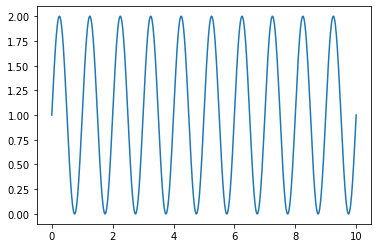The figure contains all the object representing axes, graphics, text and labels. The axes are the boundaring box with ticks and labels.

If we want to create a single figure with multiple lines

In :
x = np.arange(0.0, 2.0, 0.01)
y = 1 + np.sin(2 * np.pi * x)

x2 = np.arange(0.0, 2.0, 0.01)
y2 = x2**2

# figure and axes
fig = plt.figure()
ax = plt.axes()

# Plot
ax.plot(x, y)
ax.plot(x2,y2)

Out:
[<matplotlib.lines.Line2D at 0x7fd56b3b3b80>]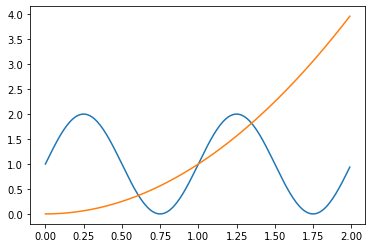In order for the graph to be interpreted it is necessary to say what we are graphing! For this let's add a label on the x and y axes and add a legend to each line. We can also give it a title.

In :
plt.plot(x, y,label='f1(x)')
plt.plot(x2,y2,label='f2(x)');
plt.xlabel('x')
plt.ylabel('y')
plt.title("Simple line plots")
plt.legend()
plt.show()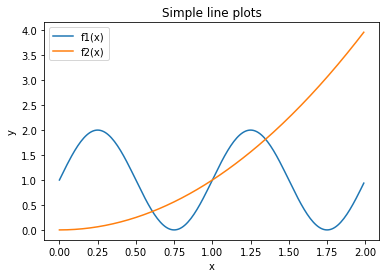The plt.plot( ) function takes additional arguments that can be used to specify the color and styles.

In :
fig = plt.figure()
ax = plt.axes()

ax.plot(x, y, color = 'black', linewidth = 4, linestyle = '-.',label='f1(x)')
plt.xlabel('x',fontweight='bold',fontsize=14)
plt.ylabel('y',fontweight='bold',fontsize=14)
plt.title("Simple line plots",fontweight='bold',fontsize=16)
# ticks styles
plt.xticks(fontweight='bold',fontsize=12)
plt.yticks(fontweight='bold',fontsize=12)
plt.legend(loc='lower right', shadow=True, fontsize=13)
plt.show()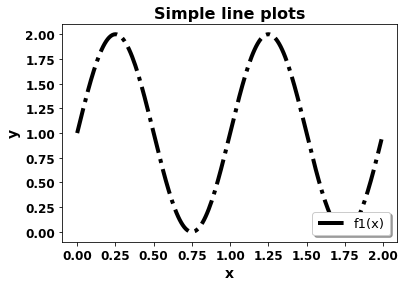Adjusting the plot

In :
fig = plt.figure()
ax = plt.axes()

ax.plot(x, y, color = 'k', linewidth = 2, linestyle = '-')

# labels and tittle
ax.set(xlabel='Time (s)', ylabel='Temperature (°C)',
title='Temperature Time Serie')

# axis limits
ax.set(xlim = (0,1), ylim = (0,2.5))

# grid
ax.grid()

# save the figure
#fig.savefig("test.png")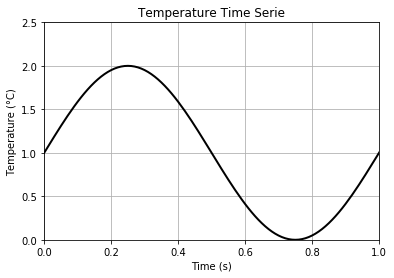#### Subplots¶

Sometimes we will want to visualize two graphs in the same figure at the same time. We can do this by defining the matplotlib subplots object.

subplots creates afig object that corresponds to the figure (the whole rectangle where we are going to graph) and several axes in the axes object, which correspond to the different subplots that we are going to make inside the figure.

In :
# difine the dataset
x1 = np.linspace(0.0, 5.0, 100)
x2 = np.linspace(0.0, 2.0, 100)

y1 = np.cos(2 * np.pi * x1) * np.exp(-x1)
y2 = np.cos(2 * np.pi * x2)

# Figure and axes
#  (2,1) represents two rows s
fig, axes = plt.subplots(2,1)
fig.suptitle('Vertical stacked axes')
axes.plot(x1, y1, color = 'k', linewidth = 2, linestyle = '-')
axes.set(xticklabels=[])# delete x tick labels
axes.plot(x2, y2, color = 'k', linewidth = 2, linestyle = '-')

Out:
[<matplotlib.lines.Line2D at 0x7fd56f4ac2b0>]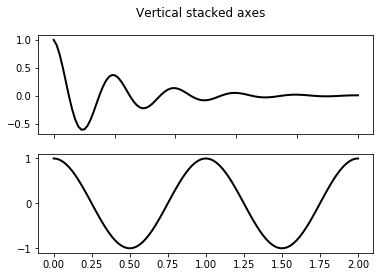More information#### Scatter plots¶

Another commonly used plot type is the simple scatter plot. The points are represented individually with a dot, circle, or other shape.

In :
from sklearn.datasets import make_blobs

X, y = make_blobs(n_samples=200, centers=3,
random_state=0, cluster_std=2)

In :
fig = plt.figure()
plt.scatter(X[:, 0], X[:, 1], c=y, s=50, cmap='jet', marker='*')
plt.xlabel('Feature x1')
plt.ylabel('Feature x2')
plt.show()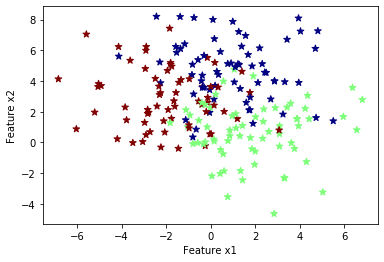Making a publication quality plot with Python

In :
fig = plt.figure()
ax = plt.axes()

ax.scatter(X[:, 0], X[:, 1],c=y,alpha = 0.3)

ax.set(xlabel='Feature x1', ylabel='Feature x1',
title='Scatter plots', xlim = (0,6))

Out:
[Text(0, 0.5, 'Feature x1'),
(0, 6),
Text(0.5, 0, 'Feature x1'),
Text(0.5, 1.0, 'Scatter plots')]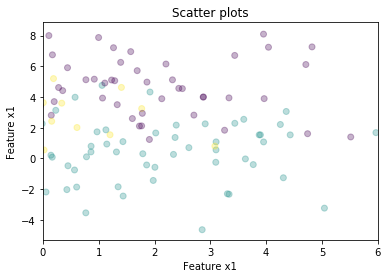## Seaborn¶

Seaborn is a Python data visualization library based on matplotlib. It provides a high-level interface for drawing attractive and informative statistical graphics.

#### Comparison between Matplotlib and Seaborn¶

We will repeat previous figure with seaborn. What is your opinion?

In :
sns.set(style="darkgrid")
ax = sns.scatterplot(X[:, 0], X[:, 1],hue=y)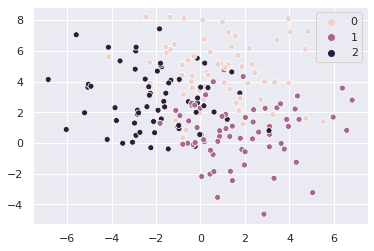Now we will make amazing figures with Seaborn using Iris dataset

Let´s import the dataset IRIS

In :
# Load an example dataset with long-form data
iris = sns.load_dataset("iris")
iris.head()

Out:
sepal_length sepal_width petal_length petal_width species
0 5.1 3.5 1.4 0.2 setosa
1 4.9 3.0 1.4 0.2 setosa
2 4.7 3.2 1.3 0.2 setosa
3 4.6 3.1 1.5 0.2 setosa
4 5.0 3.6 1.4 0.2 setosa

There is no universal best way to visualize data. Different questions are best answered by different kinds of visualizations. Seaborn tries to make it easy to switch between different visual representations that can be parameterized with the same dataset-oriented API.

In :
# Plot the responses for different events and regions
b=sns.factorplot(x='sepal_width', y='sepal_length',col='species', data=iris, alpha=.5,kind="swarm")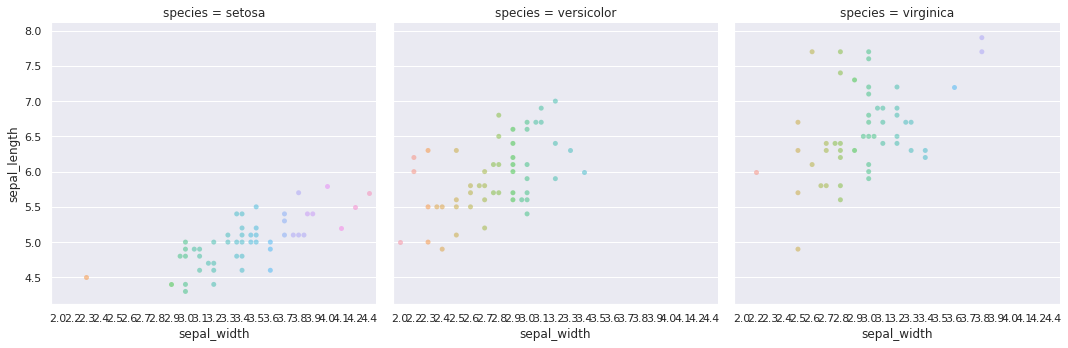Box-plot and violin plot

Violin plots are similar to box plots, except that they also show the probability density of the data at different values, usually smoothed by a kernel density estimator.

In :
h0=sns.catplot(y='sepal_width',x='species', kind='box' , data=iris)

Out:
<seaborn.axisgrid.FacetGrid at 0x7fd5680ace20>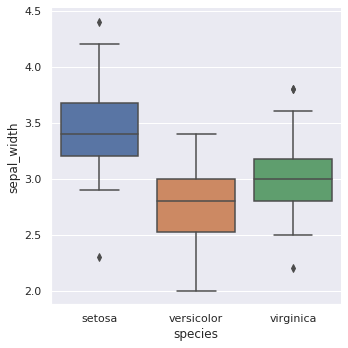In :
h1=sns.violinplot(x='species',y='petal_length',data=iris)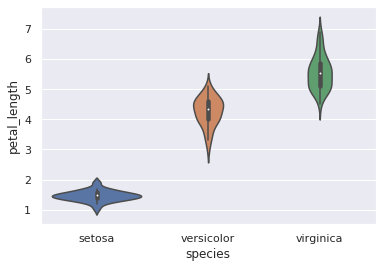Visualizing dataset structure

In :
sns.jointplot(x='sepal_width',y='petal_length',data=iris)

Out:
<seaborn.axisgrid.JointGrid at 0x7fd568a56ee0>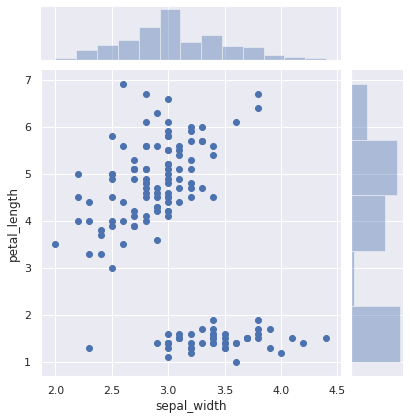More information